• 引言 学习神经网络的时候我们总是听到激活函数这个词，而且很多资料都会提到常用...有哪些激活函数，都什么性质和特点？ 应用中如何选择合适的激活函数？ 如果你对以上几个问题不是很清楚，下面的内容对你是...
引言
学习神经网络的时候我们总是听到激活函数这个词，而且很多资料都会提到常用的激活函数，比如Sigmoid函数、tanh函数、Relu函数。那么我们就来详细了解下激活函数方方面面的知识。本文的内容包括几个部分：
什么是激活函数？激活函数的用途（为什么需要激活函数）？有哪些激活函数，都有什么性质和特点？应用中如何选择合适的激活函数？
如果你对以上几个问题不是很清楚，下面的内容对你是有价值的。
什么是激活函数？
首先要了解神经网络的基本模型。（不熟悉的同学请去看本人另外一篇介绍：人工神经网络基本原理） 单一神经元模型如下图所示。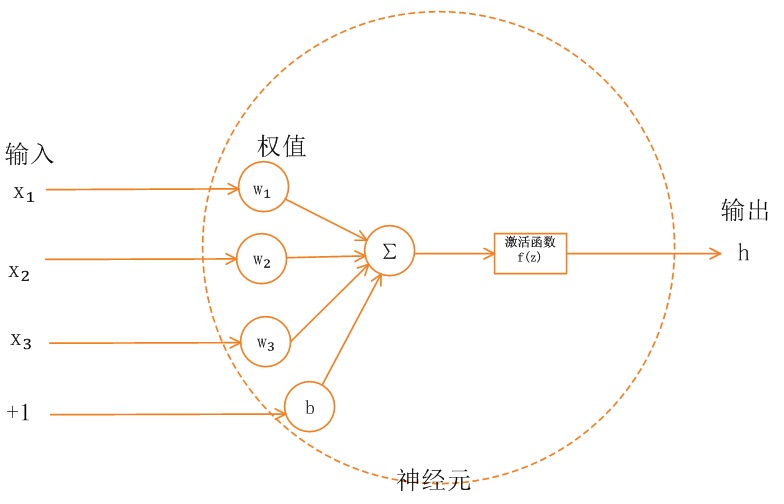神经网络中的每个神经元节点接受上一层神经元的输出值作为本神经元的输入值，并将输入值传递给下一层，输入层神经元节点会将输入属性值直接传递给下一层（隐层或输出层）。在多层神经网络中，上层节点的输出和下层节点的输入之间具有一个函数关系，这个函数称为激活函数（又称激励函数）。
激活函数的用途（为什么需要激活函数）？
如果不用激励函数（其实相当于激励函数是f(x) = x），在这种情况下你每一层节点的输入都是上层输出的线性函数，很容易验证，无论你神经网络有多少层，输出都是输入的线性组合，与没有隐藏层效果相当，这种情况就是最原始的感知机（Perceptron）了，那么网络的逼近能力就相当有限。正因为上面的原因，我们决定引入非线性函数作为激励函数，这样深层神经网络表达能力就更加强大（不再是输入的线性组合，而是几乎可以逼近任意函数）。
有哪些激活函数，都有什么性质和特点？
早期研究神经网络主要采用sigmoid函数或者tanh函数，输出有界，很容易充当下一层的输入。 近些年Relu函数及其改进型（如Leaky-ReLU、P-ReLU、R-ReLU等）在多层神经网络中应用比较多。下面我们来总结下这些激活函数：
Sigmoid函数
Sigmoid 是常用的非线性的激活函数，它的数学形式如下：

f

(

z

)

=

1

1

+

e

−

z

f(z)=\frac{1}{1+e^{-z}}

Sigmoid的几何图像如下：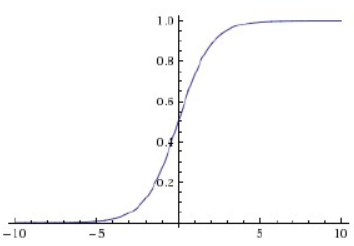特点： 它能够把输入的连续实值变换为0和1之间的输出，特别的，如果是非常大的负数，那么输出就是0；如果是非常大的正数，输出就是1. 缺点： sigmoid函数曾经被使用的很多，不过近年来，用它的人越来越少了。主要是因为它固有的一些 缺点。 缺点1：在深度神经网络中梯度反向传递时导致梯度爆炸和梯度消失，其中梯度爆炸发生的概率非常小，而梯度消失发生的概率比较大。首先来看Sigmoid函数的导数，如下图所示：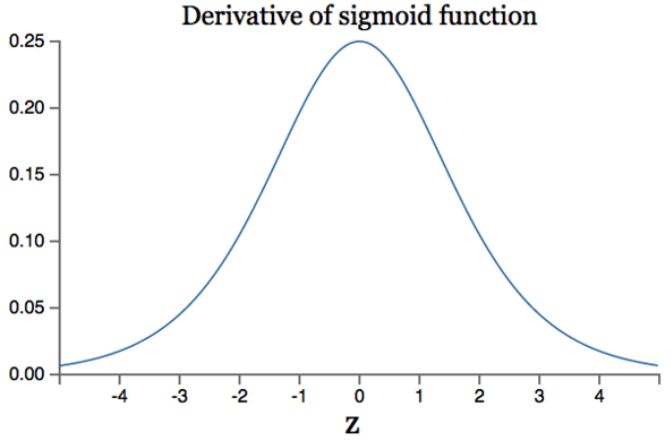如果我们初始化神经网络的权值为

[

0

,

1

]

[0,1]

之间的随机值，由反向传播算法的数学推导可知，梯度从后向前传播时，每传递一层梯度值都会减小为原来的0.25倍，如果神经网络隐层特别多，那么梯度在穿过多层后将变得非常小接近于0，即出现梯度消失现象；当网络权值初始化为

(

1

,

+

∞

)

(1,+∞)

区间内的值，则会出现梯度爆炸情况。 详细数学分析见文章：http://neuralnetworksanddeeplearning.com/chap5.html 中文译文：深度神经网络为何很难训练 缺点2：Sigmoid 的 output 不是0均值（即zero-centered）。这是不可取的，因为这会导致后一层的神经元将得到上一层输出的非0均值的信号作为输入。 产生的一个结果就是：如

x

&gt;

0

,

f

=

w

T

x

+

b

x&gt;0, \ f= w^Tx+b

,那么对w求局部梯度则都为正，这样在反向传播的过程中w要么都往正方向更新，要么都往负方向更新，导致有一种捆绑的效果，使得收敛缓慢。 当然了，如果按batch去训练，那么那个batch可能得到不同的信号，所以这个问题还是可以缓解一下的。因此，非0均值这个问题虽然会产生一些不好的影响，不过跟上面提到的梯度消失问题相比还是要好很多的。 缺点3：其解析式中含有幂运算，计算机求解时相对来讲比较耗时。对于规模比较大的深度网络，这会较大地增加训练时间。
tanh函数
tanh函数解析式：

t

a

n

h

(

x

)

=

e

x

−

e

−

x

e

x

+

e

−

x

tanh(x)=\frac{e^{x}-e^{-x}}{e^{x}+e^{-x}}

tanh函数及其导数的几何图像如下图：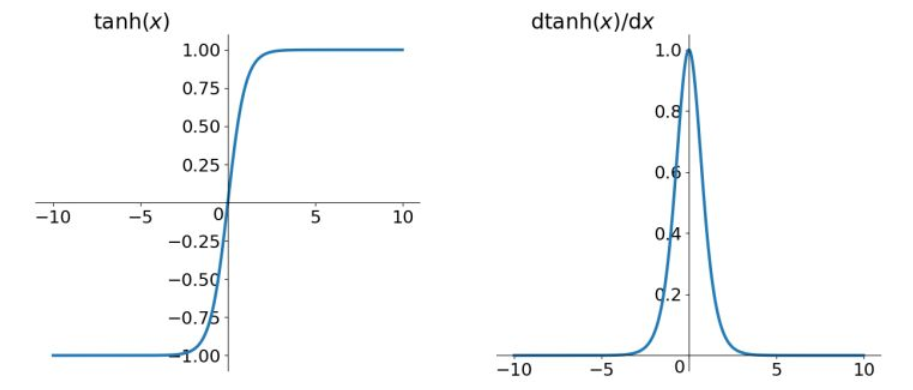tanh读作Hyperbolic Tangent，它解决了Sigmoid函数的不是zero-centered输出问题，然而，梯度消失（gradient vanishing）的问题和幂运算的问题仍然存在。
Relu函数
Relu函数的解析式：

R

e

l

u

=

m

a

x

(

0

,

x

)

Relu=max(0,x)

Relu函数及其导数的图像如下图所示：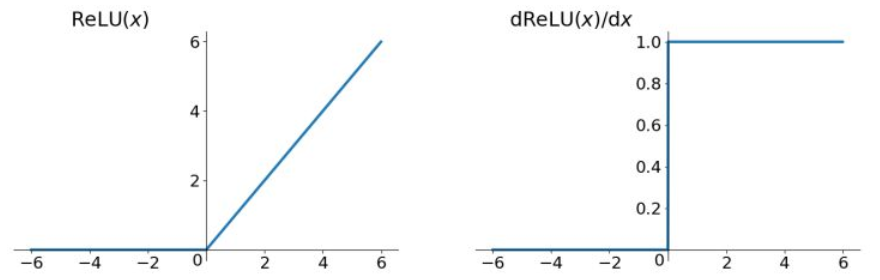ReLU函数其实就是一个取最大值函数，注意这并不是全区间可导的，但是我们可以取sub-gradient，如上图所示。ReLU虽然简单，但却是近几年的重要成果，有以下几大优点： 1） 解决了gradient vanishing问题 (在正区间) 2）计算速度非常快，只需要判断输入是否大于0 3）收敛速度远快于sigmoid和tanh
尽管存在这两个问题，ReLU目前仍是最常用的activation function，在搭建人工神经网络的时候推荐优先尝试！
Leaky ReLU函数（PReLU）
函数表达式：

f

(

x

)

=

m

a

x

(

α

x

,

x

)

f(x)=max(\alpha x,x)

Leaky Relu函数及其导数的图像如下图所示： （有同学在评论中反映下图有误，其实没有错误，左半边直线斜率非常接近0，所以看起来像是平的。就不改了，

α

=

0.01

\alpha=0.01

看起来就是这样的。感谢大家提意见 ^ _ ^）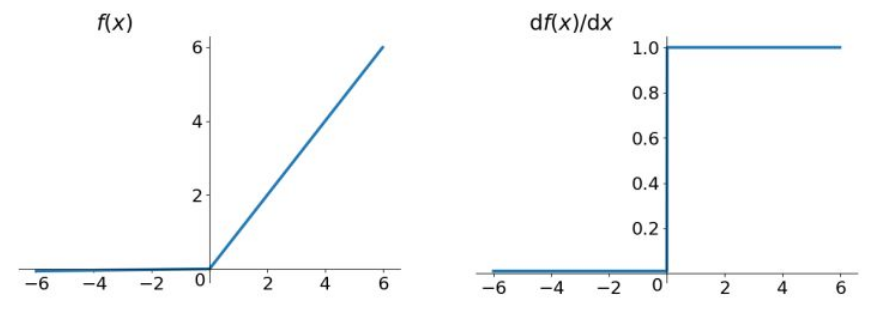人们为了解决Dead ReLU Problem，提出了将ReLU的前半段设为

α

x

\alpha x

而非0，通常

α

=

0.01

\alpha=0.01

。另外一种直观的想法是基于参数的方法，即

P

a

r

a

m

e

t

r

i

c

R

e

L

U

:

f

(

x

)

=

max

⁡

(

α

x

,

x

)

Parametric ReLU:f(x) = \max(\alpha x, x)

，其中

α

\alpha

ELU (Exponential Linear Units) 函数
函数表达式：

f

(

x

)

=

{

x

,

if

x

&gt;

0

α

(

e

x

−

1

)

,

otherwise

f(x)= \begin{cases}x,&amp; \text{if } x &gt; 0\\ \alpha(e^x - 1), &amp; \text{otherwise} \end{cases}

函数及其导数的图像如下图所示：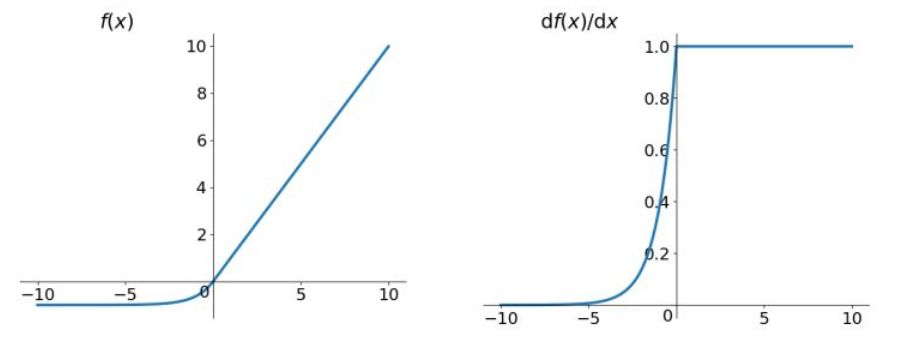ELU也是为解决ReLU存在的问题而提出，显然，ELU有ReLU的基本所有优点，以及：
不会有Dead ReLU问题
输出的均值接近0，zero-centered

它的一个小问题在于计算量稍大。类似于Leaky ReLU，理论上虽然好于ReLU，但在实际使用中目前并没有好的证据ELU总是优于ReLU。
MaxOut函数
这个函数可以参考论文《maxout networks》，Maxout是深度学习网络中的一层网络，就像池化层、卷积层一样等，我们可以把maxout 看成是网络的激活函数层，我们假设网络某一层的输入特征向量为：X=（x1,x2,……xd），也就是我们输入是d个神经元。Maxout隐藏层每个神经元的计算公式如下：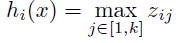上面的公式就是maxout隐藏层神经元i的计算公式。其中，k就是maxout层所需要的参数了，由我们人为设定大小。就像dropout一样，也有自己的参数p(每个神经元dropout概率)，maxout的参数是k。公式中Z的计算公式为：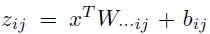权重w是一个大小为(d,m,k)三维矩阵，b是一个大小为(m,k)的二维矩阵，这两个就是我们需要学习的参数。如果我们设定参数k=1，那么这个时候，网络就类似于以前我们所学普通的MLP网络。 我们可以这么理解，本来传统的MLP算法在第i层到第i+1层，参数只有一组，然而现在我们不这么干了，我们在这一层同时训练n组的w、b参数，然后选择激活值Z最大的作为下一层神经元的激活值，这个max（z）函数即充当了激活函数。
应用中如何选择合适的激活函数？
这个问题目前没有确定的方法，凭一些经验吧。 1）深度学习往往需要大量时间来处理大量数据，模型的收敛速度是尤为重要的。所以，总体上来讲，训练深度学习网络尽量使用zero-centered数据 (可以经过数据预处理实现) 和zero-centered输出。所以要尽量选择输出具有zero-centered特点的激活函数以加快模型的收敛速度。 2）如果使用 ReLU，那么一定要小心设置 learning rate，而且要注意不要让网络出现很多 “dead” 神经元，如果这个问题不好解决，那么可以试试 Leaky ReLU、PReLU 或者 Maxout. 3）最好不要用 sigmoid，你可以试试 tanh，不过可以预期它的效果会比不上 ReLU 和 Maxout.
参考资料
1.聊一聊深度学习的activation function—夏飞 2.http://blog.csdn.net/cyh_24/article/details/50593400 3.http://www.cnblogs.com/tornadomeet/p/3428843.html 4.《maxout networks》
展开全文激活函数 激励函数 人工智能 神经网络
• ## 常用激活函数

千次阅读 2019-07-20 11:32:46
今天这篇文章对一些常用激活函数做一下总结吧。在神经网络中激活函数还是很重要的，并且熟悉激活函数对理解别的问题也是很帮助的。 为什么需要激活函数 从数学上看，神经网络是一个多层复合函数。引入激活函数是...


文章目录
前言为什么需要激活函数什么样的函数可以做激活函数什么样的函数是好的激活函数常用激活函数sigmoidtanhReLULeaky ReLURandomized Leaky ReLUMaxout
参考文章

前言
今天这篇文章对一些常用激活函数做一下总结吧。在神经网络中激活函数还是很重要的，并且熟悉激活函数对理解别的问题也是很有帮助的。
为什么需要激活函数
从数学上看，神经网络是一个多层复合函数。引入激活函数是为了保证神经网络的非线性。因为线性函数无论怎样复合结果还是线性的，所以对激活函数最基本的要求是非线性的。 假设神经网络的输入是n维向量x，输出是m维向量y，它实现了如下向量到向量的映射：

R

n

→

R

m

R^n \rightarrow R^m

我们将这个函数记为

y

=

h

(

x

)

y = h(x)

神经网络第l层的变换写成矩阵和向量形式为：

u

(

l

)

=

W

(

l

)

x

(

l

−

1

)

+

b

(

l

)

x

(

l

)

=

f

(

u

(

l

)

)

\begin{aligned} &amp; u^{(l)} = W^{(l)}x^{(l-1)} + b^{(l)} \\ &amp; x^{(l)} = f(u^{(l)}) \end{aligned}

其中W是权重矩阵，b是偏置向量，u是临时结果，x是神经网络每一层的输入或输出。激活函数分别作用于向量u的每一个分量，产生一个向量输出x。在正向传播阶段，反复用上面的公式进行计算，最后得到网络的输出。对于一个3层的网络，整个映射可以写成：

h

(

x

)

=

f

(

W

(

3

)

f

(

W

(

2

)

(

f

(

W

(

1

)

x

+

b

(

1

)

)

+

b

(

2

)

)

+

b

(

3

)

)

h(x) = f(W^{(3)}f(W^{(2)}(f(W^{(1)}x + b^{(1)}) + b^{(2)}) + b^{(3)})

这是一个3层的复合函数。从这里可以清晰的看到，如果没有激活函数，整个函数将是一个线性函数：

W

(

3

)

(

W

(

2

)

(

W

(

1

)

x

+

b

(

1

)

)

+

b

(

2

)

)

+

b

(

3

)

W^{(3)}(W^{(2)}(W^{(1)}x + b^{(1)}) + b^{(2)}) + b^{(3)}

什么样的函数可以做激活函数
前面已经说过，为保证非线性，激活函数必须为非线性函数，但仅仅具有非线性是不够的。神经网络在本质上是一个复合函数，这会让我们思考一个问题：这个函数的建模能力有多强？即它能模拟什么样的目标函数？已经证明，只要激活函数选择得当，神经元个数足够多，使用3层即包含一个隐含层的神经网络就可以实现对任何一个从输入向量到输出向量的连续映射函数的逼近，这个结论称为万能逼近（universal approximation）定理。万能定理对激活函数的要求是必须非常数、有界、单调递增并且连续。 当然，仅仅满足万能逼近定理的要求也是不够的。神经网络的训练一般采用反向传播算法和梯度下降法。反向传播算法从复合函数求导的链式法则导出，因为神经网络是一个多层的复合函数。在反向传播时，误差项的计算公式为：

δ

(

l

)

=

(

W

(

l

+

1

)

)

T

δ

(

l

+

1

)

⊙

f

′

(

u

(

l

)

)

\delta^{(l)} = (W^{(l+1)})^T \delta^{(l+1)} \odot f&#x27;(u^{(l)})

由于使用梯度下降法需要计算损失函数对参数的梯度值，这个梯度值根据上面的误差项计算，而误差项的计算又涉及到计算激活函数的导数，因此激活函数必须是可导的。实际应用时并不要求它在定义域内处处可导，只要是几乎处处可导即可。
什么样的函数是好的激活函数
反向传播算法计算误差项时每一层都要乘以本层激活函数的导数。如果激活函数导数的绝对值小于1，多次连乘之后误差项很快会衰减到接近于0，参数的梯度值由误差项计算得到，从而导致前面层的权重梯度接近于0，参数没有得到有效更新，这称为梯度消失问题。与之相反的是梯度爆炸问题，如果激活函数的导数的绝对值大于1，多次乘积之后权重值会趋向于非常大的数，这称为梯度爆炸。 理论分析和实验结果都证明，随着网络层数的增加，反向传播的作用越来越小，网络更加难以训练和收敛。 这就引入了激活函数饱和性的概念。如果一个激活函数满足：

lim

⁡

x

→

+

∞

f

′

(

x

)

=

0

\lim_{x \to + \infty} f&#x27;(x) = 0

即在正半轴函数的导数趋于0，则称该函数为右饱和。类似的如果满足：

lim

⁡

x

→

−

∞

f

′

(

x

)

=

0

\lim_{x \to - \infty} f&#x27;(x) = 0

即在负半轴函数的导数趋于0，则称该函数左饱和。如果一个激活函数既满足左饱和又满足右饱和，称之为饱和。如果存在常数c，当

x

&gt;

c

x&gt;c

时有：

f

′

(

x

)

=

0

f&#x27;(x) = 0

则称函数右硬饱和；当

x

&lt;

c

x&lt;c

时有：

f

′

(

x

)

=

0

f&#x27;(x) = 0

则称函数左硬饱和。既满足左硬饱和又满足右硬饱和的激活函数为硬饱和函数。饱和性和梯度消失问题密切相关。在反向传播过程中，误差项在每一层都要乘以激活函数导数值，一旦x的值落入饱和区间，多次乘积之后会导致梯度越来越小，从而出现梯度消失问题。 所以，非饱和函数相比饱和函数来说更能成为好的激活函数。
常用激活函数
sigmoid
sigmoid函数的表达式为：

σ

(

x

)

=

1

1

+

e

−

x

\sigma(x) = \frac{1}{1+e^{-x}}

其函数图像如下图所示：sigmoid的值域为(0,1)。其导数为：

σ

′

(

x

)

=

σ

(

x

)

(

1

−

σ

(

x

)

)

\sigma&#x27;(x) = \sigma(x)(1 - \sigma(x))

优点
求导容易，利用其本身函数值即可。
缺点
函数具有软饱和性，容易产生梯度消失问题。输出不以0为中心，如果数据输入神经元是正的，那么会导致计算的梯度也始终为正。

e

−

x

e^{-x}

计算耗时。
tanh
tanh函数的表达式为：

t

a

n

h

(

x

)

=

e

x

−

e

−

x

e

x

+

e

−

x

=

2

σ

(

2

x

)

−

1

tanh(x) = \frac{e^x - e^{-x}}{e^x + e^{-x}} = 2 \sigma(2x) - 1

其函数图像如下图所示：tanh的值域为(-1, 1)。其导数为：

t

a

n

h

′

(

x

)

=

1

−

(

t

a

n

h

(

x

)

)

2

tanh&#x27;(x) = 1 - (tanh(x))^2

优点
输出以0为中心。比sigmoid函数训练时收敛更快。
缺点
仍然是饱和函数，没有解决梯度消失问题。
ReLU
ReLU函数的表达式为：

R

e

L

U

(

x

)

=

m

a

x

(

0

,

x

)

ReLU(x) = max(0, x)

其函数图像如下图所示：其导数为：

R

e

L

U

′

(

x

)

=

s

i

g

n

(

x

)

=

{

1

,

x

&gt;

0

,

0

,

x

&lt;

0

ReLU&#x27;(x) = sign(x) = \begin{cases} 1, &amp; x &gt; 0,\\ 0, &amp; x &lt;0 \end{cases}

需要注意的是，ReLU在原点处不可导。 优点
解决了部分梯度消失问题训练收敛速度相比tanh更快
缺点
当x<0时，出现梯度消失问题。此时相当于神经元死亡。
对于神经元死亡问题，我们简单解释一下。 假设现有损失函数为交叉熵损失函数，

y

y

为实际值，

y

^

\hat y

为预测值，那么其计算公式为：

L

(

y

,

y

^

)

=

−

(

y

l

o

g

(

y

^

)

+

(

1

−

y

)

l

o

g

(

1

−

y

^

)

)

L(y, \hat y) = -(ylog(\hat y) + (1 - y)log(1-\hat y))

如果正例为1，负例为0，那么当

y

^

=

y

\hat y = y

时，损失函数值为0；当

y

^

=

0

\hat y = 0

而

y

=

1

y = 1

时，损失函数值为无穷大；当

y

^

=

1

\hat y = 1

而

y

=

0

y = 0

时，损失函数值为无穷大。 假设神经网络某层的计算公式为：

y

=

R

e

L

U

(

W

T

X

+

b

)

y = ReLU(W^T X + b)

如果学习率设置的比较大，且此时的实际值为负例，训练结果为使模型往实际值拟合，那么权重W就会突然变得很小，使

W

T

X

+

b

W^T X + b

Leaky ReLU
Leaky ReLU函数的表达式：

f

(

x

)

=

{

x

,

x

&gt;

0

,

α

x

,

x

≤

0

f(x) = \begin{cases} x, &amp; x &gt; 0, \\ \alpha x, &amp; x \le 0 \end{cases}

式中

α

\alpha

是一个很小的正数，比如0.01。 其函数图像如下图所示：其导数为：

f

′

(

x

)

=

{

1

,

x

&gt;

0

,

α

,

x

≤

0

f&#x27;(x) = \begin{cases} 1, &amp; x&gt;0, \\ \alpha, &amp; x \le 0 \end{cases}

优点
解决了ReLU神经元死掉的问题。
比Leaky ReLU更进一步的是PReLU，它与Leaky ReLU的区别是，其计算公式中的

α

\alpha

不是预先定义好的，而是被当做参数去训练。
Randomized Leaky ReLU
Randomized Leaky ReLU中的

α

\alpha

在训练阶段是从高斯分布

U

(

l

,

u

)

U(l, u)

中随机取出来的，然后在测试过程中进行纠正。 其表达式为：

y

j

i

=

{

x

j

i

,

x

j

i

≥

0

,

α

j

i

x

j

i

,

x

j

i

&lt;

0

where

α

j

i

∼

U

(

l

,

u

)

,

l

&lt;

u

and

l

,

u

∈

[

0

,

1

)

y_{ji} = \begin{cases} x_{ji}, &amp; x_{ji} \ge 0, \\ \alpha_{ji}x_{ji}, &amp; x_{ji} &lt; 0 \end{cases} \\ \text{where }\alpha_{ji} \sim U(l, u), l &lt; u \text{ and } l, u \in [0, 1)

其函数图像如下图所示：Maxout
Maxout函数表达式如下：

f

i

(

x

)

=

m

a

x

j

∈

[

1

,

k

]

z

i

j

f_i(x) = max_{j \in [1, k]} z_{ij}

假设w是2维的，那么有：

f

(

x

)

=

m

a

x

(

w

1

T

X

+

b

1

,

w

2

T

X

+

b

2

)

f(x) = max({w_1}^TX+b_1, {w_2}^TX+b_2)

ReLu和Leaky ReLU都是它的变形（比如,

w

1

,

b

1

=

0

w_1, b_1 = 0

的时候，就是ReLU ）。 Maxout的拟合能力是非常强的，它可以拟合任意的凸函数，最直观的解释就是任意的凸函数都可以由分段线性函数以任意精度拟合，前提是“隐隐含层”的神经元个数可以任意多。 因为Maxout可以拟合任意凸函数，所以其没有固定的函数图像，下图是其一些拟合示例：优点
计算简单。解决了梯度消失问题，且不会产生死亡神经元。训练收敛速度快。
缺点
每个神经元的参数加倍，导致参数数量激增。
其实还有很多其他的激活函数，限于篇幅这里就不一一分析了。
参考文章
理解神经网络的激活函数 【神经网络】激活函数面面观 常用激活函数的比较 为什么Relu会导致死亡节点 Maxout激活函数原理及实现
展开全文• 机器学习常用激活函数   摘要： 激活函数就是神经网络输出端的一个节点，来连接两个神经网络。本文主要介绍激活函数的功能以及激活函数类型。 什么是激活函数？ 激活函数就是神经网络输出端的一个节点，来...
机器学习常用激活函数

摘要： 激活函数就是神经网络输出端的一个节点，来连接两个神经网络。本文主要介绍激活函数的功能以及激活函数类型。
什么是激活函数？
激活函数就是神经网络输出端的一个节点，来连接两个神经网络。

为什么要在神经网络中使用激活函数
激活函数用来确定神经网络的输入，比如“是”或“否”，将结果映射为[0，1]或[-1，1]之间，根据函数的曲线，可分为两种类型：线性激活函数、非线性激活函数。
1.线性激活函数（恒等激活函数）
如下图所示，函数是线性的，函数的输出范围为（-∞，+∞）。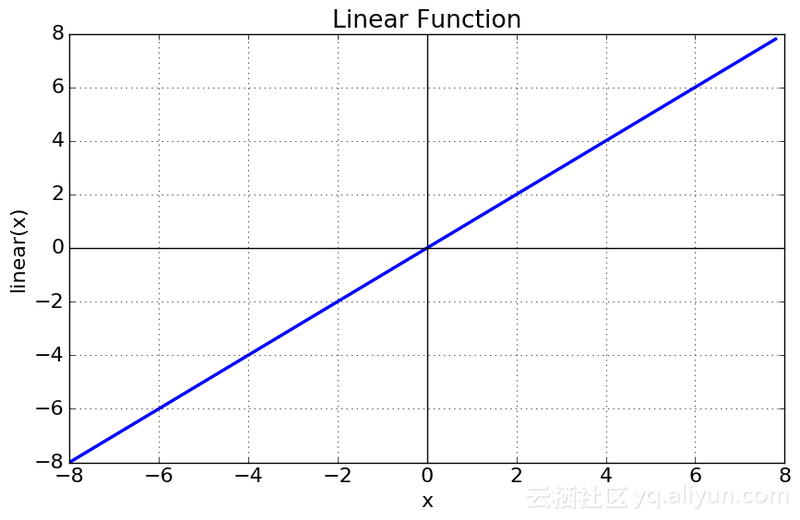线性激活函数
方程式：f(x) = x
取值范围：（-∞，+∞）

2.非线性激活函数
非线性激活函数是最常用的激活函数，其曲线如下图所示：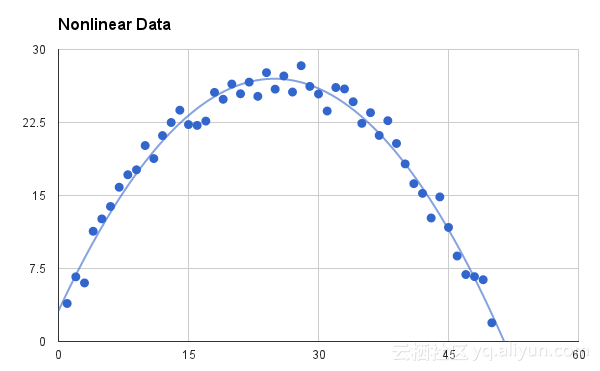非线性激活函数
使用非线性激活函数，模型可以更容易进行自我调整，并区分不同的输出。非线性激活函数中的主要术语有：
1.导数或微分：y轴随x轴的变化，称为斜率。
2.单调函数：完全递增或完全递减的函数。

根据取值范围，非线性激活函数可分为以下几种：Sigmoid激活函数、Tanh激活函数、ReLU激活函数、
1.Sigmoid激活函数（Logistic激活函数）
Sigmoid激活函数的曲线呈“S”形。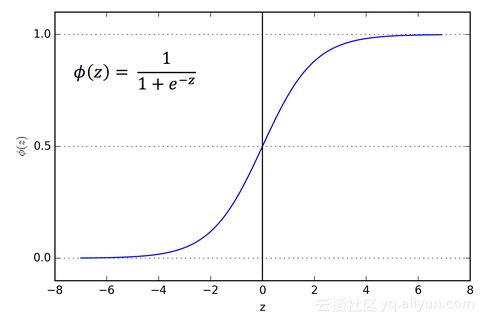Sigmoid激活函数
sigmoid函数很受大众的欢迎，其主要原因是：它的输出处于[0,1]范围内，特别适用于输出概率的模型。由于任何概率的取值在0和1范围之间，因此，sigmoid激活函数是最好的选择。

该函数是可微的，也就是说，我们可以得到“S”曲线上任意两点之间的斜率。这个函数是单调的，但是其导数不是，sigmoid 激活函数可能会导致神经网络在训练的时候卡住。
Softmax函数是一种更通用的逻辑激活函数，用于多类分类。

2.Tanh激活函数
tanh激活函数和sigmoid激活函数类似，但是要比sigmoid激活函数好。tanh激活函数的取值范围是（-1,1），曲线也呈“S”形。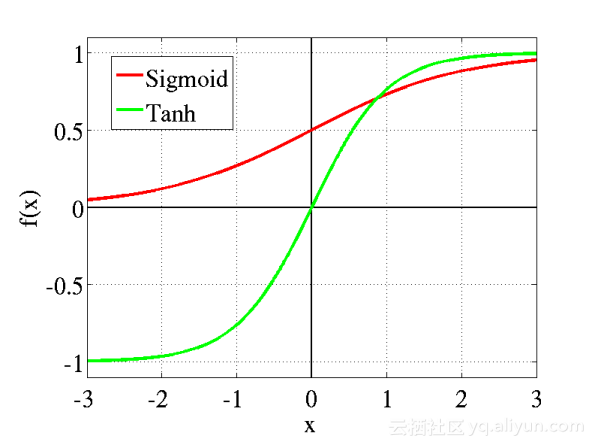sigmoid激活函数和tanh激活函数

tanh激活函数的优点在于，如果输入为负数，则输出也为负数，输入为0，则输出也近似为0。
该函数是可微分、单调的，但其导数不单调。tanh激活函数主要用于分类。
tanh和sigmoid激活函数都可用于前馈网络。

3.ReLU激活函数(Rectified Linear Unit)
在神经网络中，使用最多的激活函数是ReLU激活函数，它几乎可用于所有卷积神经网络或深度学习中。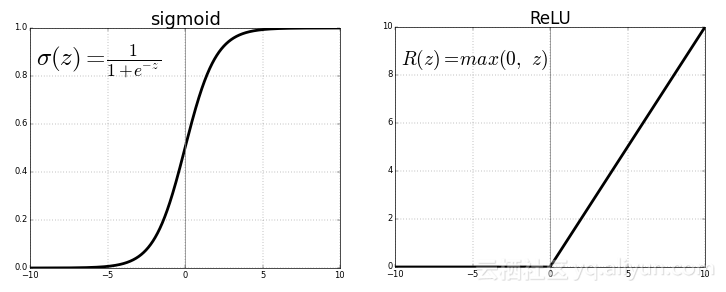ReLU激活函数和Sigmoid激活函数

如上图所示，在ReLU激活函数中，当z<0时，f(z)=0；当z>0时，f(z)=z。取值范围为[0,+∞]
ReLU激活函数及其导数都是单调的。
但这存在一个问题：当输入为负值时，输出立刻变为0，这就降低了模型拟合或训练数据的能力。反过来说，为了不影响结果，就不能映射负值输入。

4. Leaky ReLU激活函数
Leaky ReLU激活函数的出现，试图解决ReLU激活函数中出现的死亡问题。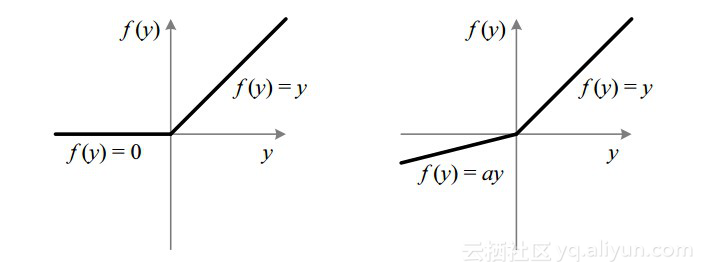ReLU激活函数和Leaky ReLU激活函数

Leaky ReLU激活函数扩大了ReLU激活函数的取值范围，如上图所示，通常，a的值为0.01左右。取值范围：（-∞，+∞）。
当a不是0.01时，该函数称为Randomized ReLU。
本质上来说，Leaky  ReLU函数和Randomized ReLU函数都是单调的。 而且，它们的导数也单调。

为什么要使用导数和微分？
在更新曲线时，我们要知道哪个方向上会发生变化，或者是根据斜率来更新曲线。这就是我们要在机器学习和深度学习的每个部分都使用微分的原因。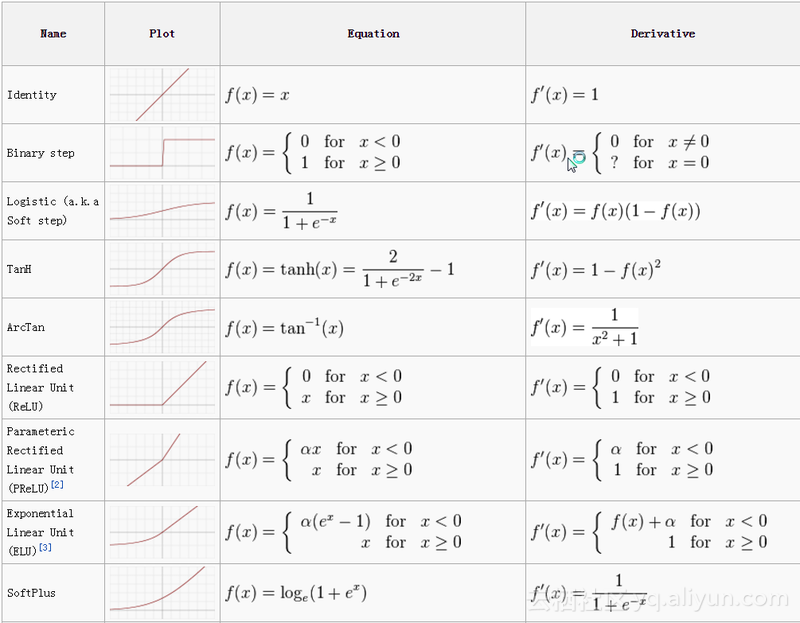激活函数汇总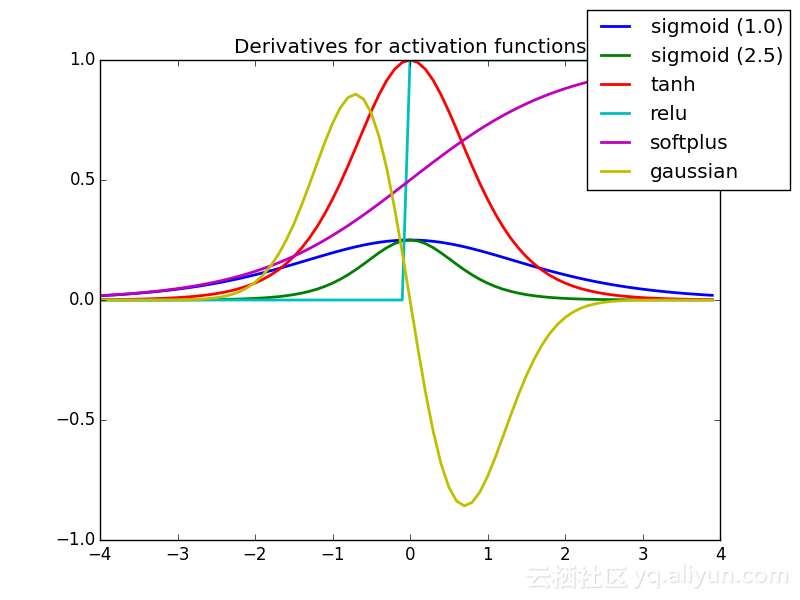激活函数的导数曲线图汇总


展开全文机器学习 Tanh
• ## PyTorch常用激活函数

万次阅读 多人点赞 2019-05-27 08:34:46
两个或多个线性网络层叠加，并不能学习一个新的东西，简单的堆叠网络层，而不经过非线性激活函数激活，学到的仍然是线性关系。 通过激活函数的加入可以学到非线性的关系，这对于特征提取具有更强的能力...
神经网络只是由两个或多个线性网络层叠加，并不能学到新的东西，简单地堆叠网络层，不经过非线性激活函数激活，学到的仍然是线性关系。
但是加入激活函数可以学到非线性的关系，就具有更强的能力去进行特征提取。

构造数据
import torch
import torch.nn.functional as F

import matplotlib.pyplot as plt

x = torch.linspace(-5, 5, 200)   # 构造一段连续的数据
x = Variable(x)	  # 转换成张量
x_np = x.data.numpy()	# 换成 numpy array, 出图时用

Relu
表达式：

r

(

x

)

=

m

a

x

(

0

,

x

)

r(x )=max(0, x)

代码：
y_relu = F.relu(x).data.numpy()
plt.plot(x_np, y_relu, c='red', label='relu')
plt.ylim((-1, 5))
plt.legend(loc='best')

plt.show()

形状如图：Sigmoid
表达式：

σ

(

z

)

=

1

1

+

e

−

x

\sigma(z)=\frac{1}{1+e^{-x}}

代码：
y_sigmoid = F.sigmoid(x).data.numpy()
plt.plot(x_np, y_sigmoid, c='red', label='sigmoid')
plt.ylim((-0.2, 1.2))
plt.legend(loc='best')

plt.show()

形状如图：Tanh
表达式：

t

a

n

h

(

x

)

=

e

x

−

e

−

x

e

x

+

e

−

x

tanh(x)=\frac{e^{x}-e^{-x}}{e^{x}+e^{-x}}

代码：
y_tanh = F.tanh(x).data.numpy()
plt.plot(x_np, y_tanh, c='red', label='tanh')
plt.ylim((-1.2, 1.2))
plt.legend(loc='best')

plt.show()

形状如图：Softplus
表达式：

ζ

(

x

)

=

l

o

g

(

1

+

e

x

)

\zeta(x)=log(1+e^x)

代码：
y_softplus = F.softplus(x).data.numpy()
plt.plot(x_np, y_softplus, c='red', label='softplus')
plt.ylim((-0.2, 6))
plt.legend(loc='best')

plt.show()

形状如图：展开全文PyTorch
• 激活函数 Logistic Softmax Relu 损失函数损失函数 代价函数
• 【神经网络】激活函数的作用及常用激活函数ReLu、Sigmoid神经网络 人工智能
• ## 常用激活函数比较

万次阅读 2017-05-10 15:27:25
常用激活函数比较 本文结构： 什么是激活函数为什么要用都什么sigmoid ，ReLU， softmax 的比较如何选择 1. 什么是激活函数 如下图，在神经元中，输入的 inputs 通过加权，求和后，还被作用了一个函数，这个...
• ## 常用激活函数介绍

千次阅读 2017-10-15 19:05:51
为什么在神经网络中需要用到激活函数呢，而且是用非线性激活函数呢，原因很简单，假如我们用的是线性激活函数的话，假设在多层神经网络，为某个神经元的计算结果，为使用线性激活函数后的结果.  则相当于使用了...tanh Relu 神经网络
• 在感知器模型、神经网络模型、深度学习模型中均会看见激活... 常用激活函数有：线性函数、斜坡函数、阶跃函数、符号函数、Sigmoid函数、双曲正切函数、Softplus函数、Softsign函数、Relu函数及其变形、Maxout函数等。深度学习 神经网络 Maxout ReLU
• ## 常用激活函数总结

千次阅读 2020-06-16 14:26:08
激活函数总结1. Sigmoid2. tanh3. ReLU4. LeakyReLU, PReLU（Parametric Relu）, RReLU 写在前面： 神经网络为什么需要激活函数： 首先数据的分布绝大多数是非线性的，而一般神经网络的计算是线性的，引入激活函数...神经网络
• 本文主要介绍深度学习常用激活函数，介绍了sigmoid激活函数，tanh激活函数，relu激活函数等relu 深度学习
• 本资源通过作者查阅资料并进行相应的修改，得到此最终版本的学习资源，是深度学习里面一些常用激活函数和损失函数对应的代码，供广大用户参考。
• ## 常用激活函数图像

千次阅读 2018-05-31 13:16:08
激励函数有很多, 不过我们平时要用到的就这几个. relu, sigmoid, tanh, softplus. import torch import torch.nn.functional as F # 激励函数都在这 from torch.autograd import Variable # 做一些假数据来观看...mat可视化
• 本文为 CS231n 中关于激活函数部分的笔记。 激活函数（Activation Function）能够把输入的特征保留并映射下来。 Sigmoid Sigmoid 非线性函数将输入映射到 (0,1)(0,1) 之间。它的数学公式为：σ(x)=11+e...深度学习
• ## 常用激活函数求导

千次阅读 2018-07-27 15:28:01
知乎：https://zhuanlan.zhihu.com/albertwang 微信公众号：AI-Research-Studio ​ ​​ 下面是赞赏码
• 激活函数1. 常用的激活函数2. 激活函数计算公式及其可视化2.1 Sigmoid...表1 常用激活函数操作 名称 层对应的类 功能 S型激活函数 torch.nn.Sigmoid Sigmoid激活函数 双曲正切函数 torch.nn.Tanh Tanh激活函神经网络 深度学习
• 本资源通过对深度学习中常用的一些激活函数和损失函数通过查阅资料进行集中总结，供其他用户参考学习，为其他用户节约相应的收集时间。深度学习
• ## TensorFlow2.0常用激活函数

千次阅读 热门讨论 2020-01-24 10:30:18
激活函数是神经网络中一个十分重要的组成部分，它可以对神经元的接收信息进行非线性变换，将变换后的信息输出到下一层神经元 激活函数给神经元引入了非线性因素，使得神经网络可以任意逼近任何非线性函数，这样神经...tensorflow python
• 在处理简单的线性可分的数据集的时候我们不需要用到激活函数仅仅依靠线性分类器就可以解决问题，但是实际生活中的绝大多数的场景并不是这样简单的，那么简单的线性分类器就没有办法起到很好的效果了，此时常用的处理...
• 激活函数（Activation Function）一般用于神经网络的层与层之间，将上一层的输出转换之后输入到下一层。如果没有激活函数引入的非线性特性，那么神经网络就只相当于原始感知机的矩阵相乘。   一、激活函数的性质 ...
• 4.4 常用激活函数讲解 神经网络的激活函数其实很多种，在前面的章节中我们介绍过两种激活函数，sign函数和purelin函数。sign函数也称为符号函数，因为sign(x)中x＞0，函数结果为1；sign(x)中x，函数结果为-1。...深度学习...